# DIY Zambretti Weather Forecaster on VFD Display

Weather forecaster, which displays the weather forecast on the screen in the form of descriptive text.

BeginnerFull instructions provided1,201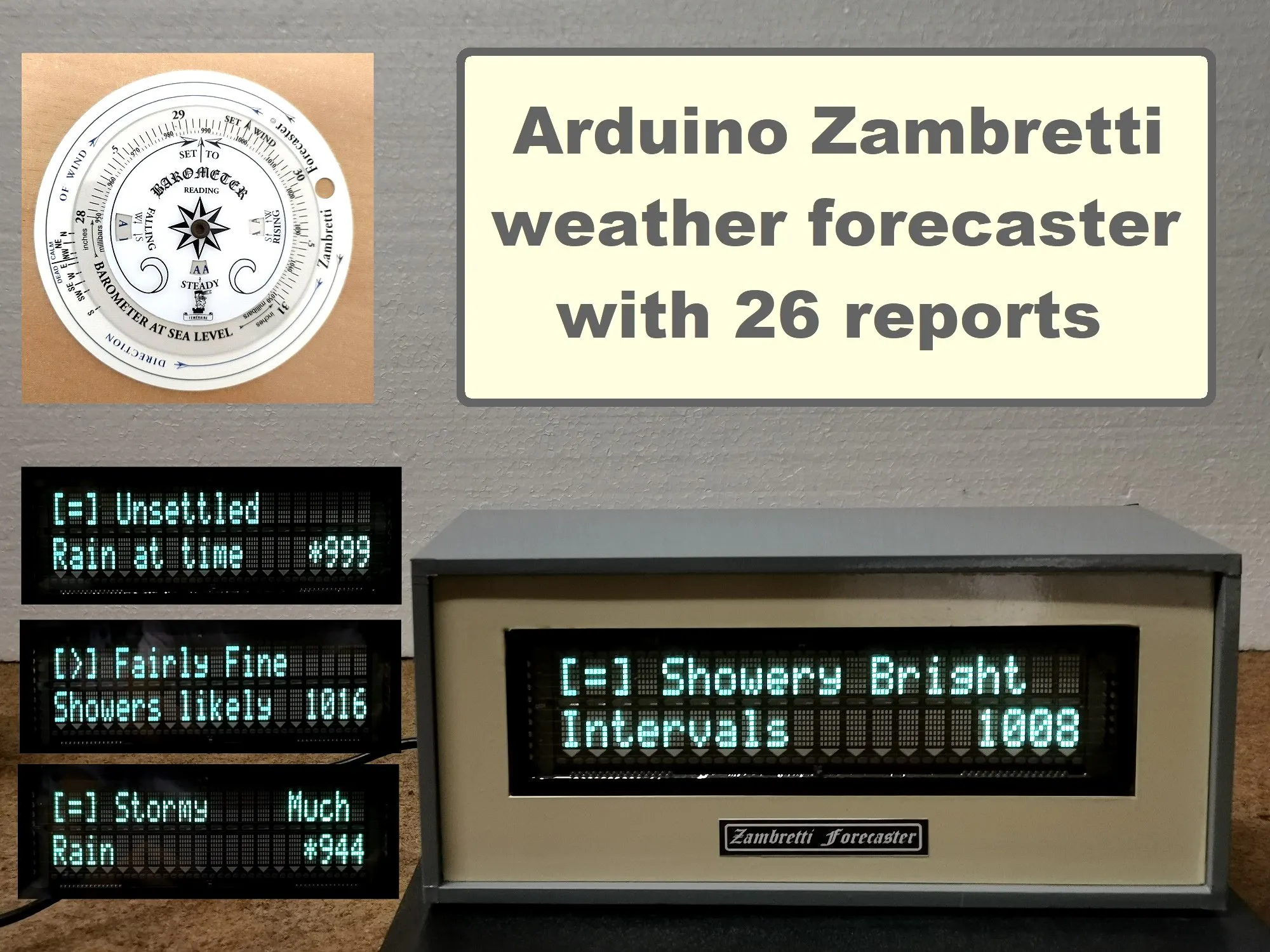## Things used in this project

### Hardware componentsArduino Nano R3
×1
 VFD Display with 20x2 characters
×1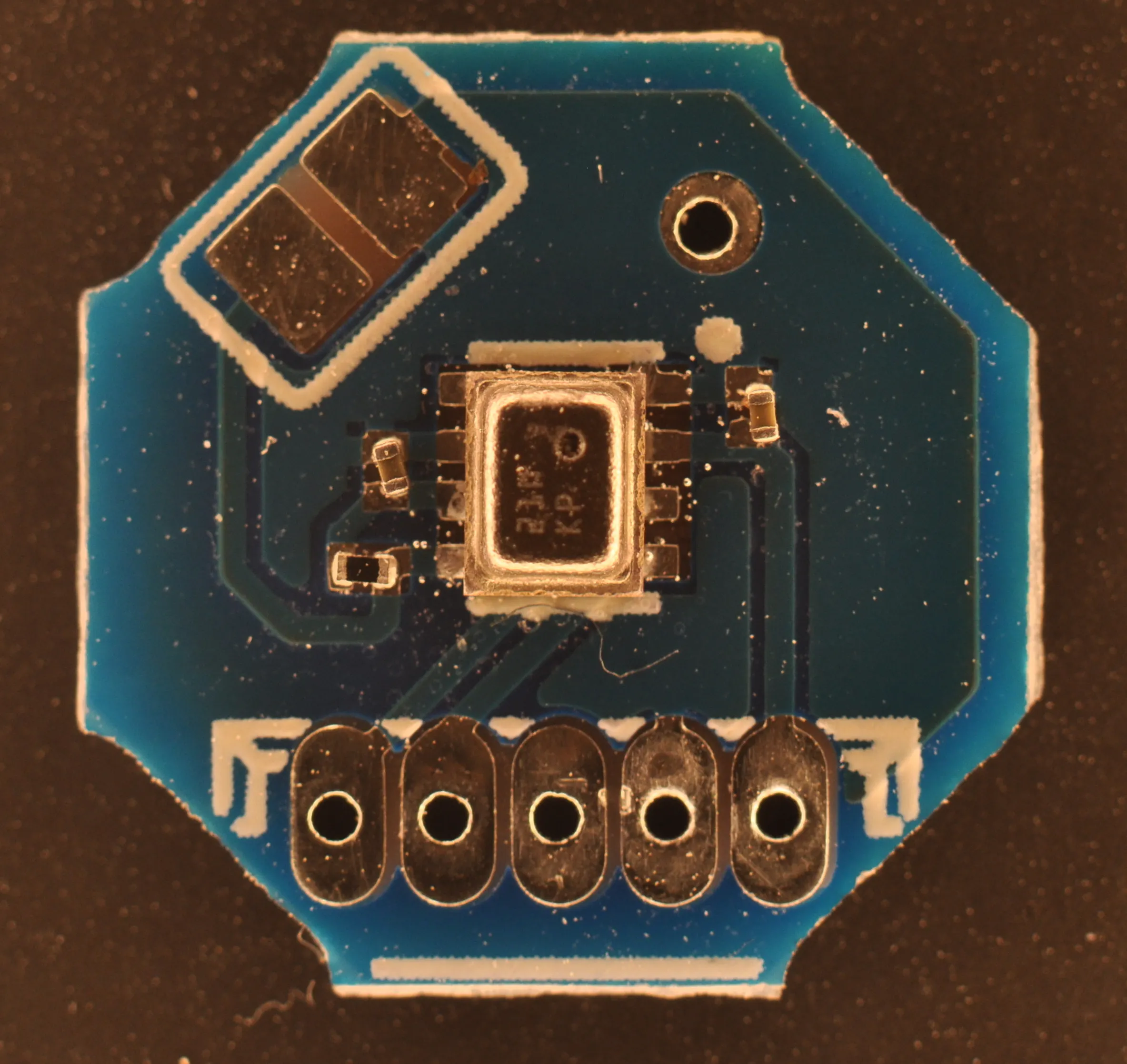MyOctopus i2c Barometric Air Pressure Sensor BMP280
×1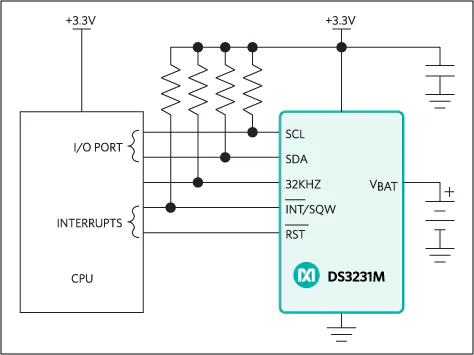Maxim Integrated DS3231M - ±5ppm, I2C Real-Time Clock
×1

### Software apps and online servicesArduino IDE

### Hand tools and fabrication machinesSoldering iron (generic)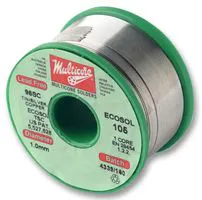## Schematics

### Schematic diagram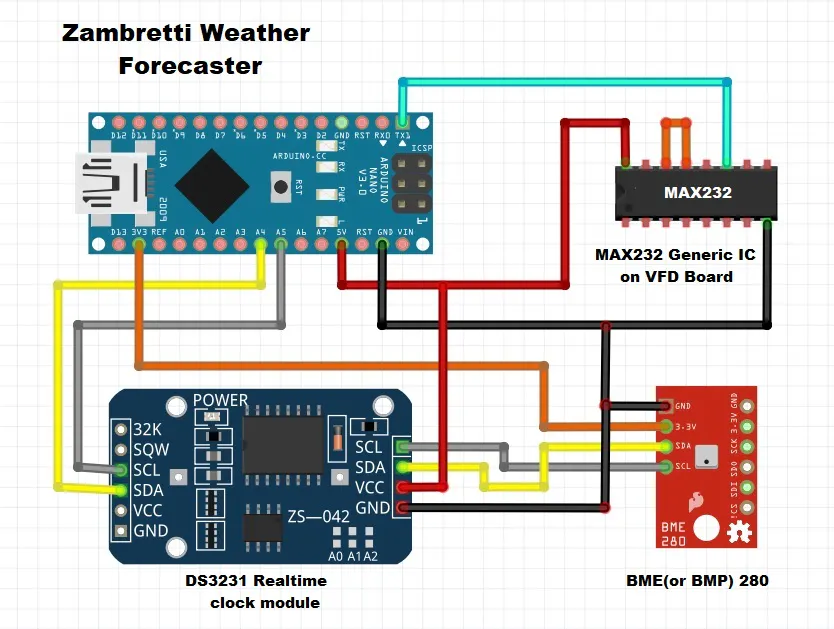## Code

### Arduino Code

C/C++
```#include <Wire.h>

#include "RTClib.h"

#define SEALEVELPRESSURE_HPA (1013.25)

RTC_DS3231 rtc;   // initialise the RTC DS3231
Adafruit_BMP280 BMP;  // initialise the BMP280 sensor

unsigned long delayTime;  // refresh rate for the readings
int t_hour = 0;
int t_minute = 0;
//int t_second =0;

int pressureArray = {0};  // here we store the pressure readings
byte counter = 0;
byte delta_time = 0;
int Z = 0;

char tStr;
char pStr;
char hStr;
char pseaStr;
char timeStr;
char dateStr;
char zambretti = "N/A";
char pressureHistory;
char pressureHistory2;

int temperature;
int humidity;
int pressure;
int altitude = 724;   // Here you should put the REAL altitude of the iGnome

void setup() {
bool status;
Serial.begin(4800);   // default settings

Serial.print(" Zambretti Weather      forecaster      ");
delay(5000);
if (! rtc.begin()) {
Serial.write("Couldn't find RTC");
while (1);
}

if (rtc.lostPower()) {
Serial.write("RTC lost power, lets set the time!");
// following line sets the RTC to the date & time this sketch was compiled

}
status = BMP.begin(0x76);
if (!status) {
while (1);
}
delayTime = 20000;

}

void loop() {

// Let's see the reading that will determine the forecast

// Serial.print("Measured altitude ");

int seapressure = station2sealevel(pressure, altitude, temperature);

DateTime now = rtc.now();
int t_hour2 = now.hour();
int t_minute2 = now.minute();
// int t_second2 = now.second();

if (t_hour2 != t_hour or t_minute2 != t_minute) {
delta_time++;
if (delta_time > 10) {    // every minute we increment delta_time, then every 10 minutes
delta_time = 0;         // we store the value in the array

if (counter == 10)  // if we read 10 values and filled up the array, we shift the array content
{
for (int i = 0; i < 9; i++) {   // we shift the array one position to the left
pressureArray[i] = pressureArray[i + 1];
}
pressureArray[counter - 1] = seapressure;
}
else {        // this code fills up the pressure array value by value until is filled up
pressureArray[counter] = seapressure;
counter++;
}
}

Z = calc_zambretti((pressureArray + pressureArray + pressureArray) / 3, (pressureArray + pressureArray + pressureArray) / 3, now.month());

// From here is just for debug purposes on the serial monitor
/*
sprintf(zambretti, "Z=%d", Z);
sprintf(tStr, "%d C", temperature);
sprintf(hStr, "%d %%", humidity);
sprintf(pStr, "%d hPa", pressure);
sprintf(pseaStr, "%d hPa", seapressure);
sprintf(dateStr, "%02d.%02d.%d", now.day(), now.month(), now.year());
sprintf(timeStr, "%02d:%02d", now.hour(), now.minute());
sprintf(pressureHistory, "%d,%d,%d,%d,%d,%d,%d,%d,%d,%d,", pressureArray, pressureArray, pressureArray,
pressureArray, pressureArray, pressureArray, pressureArray, pressureArray, pressureArray, pressureArray);

Serial.write(zambretti);
Serial.write(tStr);
Serial.write(hStr);
Serial.write(pStr);
Serial.write(pseaStr);
Serial.write(dateStr);
Serial.write(timeStr);
Serial.write(pressureHistory);
Serial.write(pressureHistory2);
*/
// END of testing purposes

if (pressureArray > 0 and pressureArray > 0) {
if (pressureArray + pressureArray + pressureArray - pressureArray - pressureArray - pressureArray >= 3) {
//RAISING
Serial.print("[>] ");
if (Z<2){
Serial.write("Settled Fine                    ");
}
else if (Z>1 and Z<=2){
Serial.write("Fine Weather                    ");
}
else if (Z>2 and Z<=3){
Serial.write("Becoming Fine                   ");
}
else if (Z>3 and Z<=4){
Serial.write("Fine Becoming   Less Settled    ");
}
else if (Z>4 and Z<=5){
Serial.write("Fine  Possibly   Showers        ");
}
else if (Z>5 and Z<=6){
Serial.write("Fairly Fine     Improving       ");
}
else if (Z>6 and Z<=7){
Serial.write("Fairly Fine Pos.Showers, early  ");
}
else if (Z>7 and Z<=8){
Serial.write("Fairly Fine     Showery Later   ");
}
else if (Z>8 and Z<=9){
Serial.write("Showery Early   Improving       ");
}
else if (Z>9 and Z<=10){
Serial.write("Changeable      Mending         ");
}
else if (Z>10 and Z<=11){
Serial.write("Fairly Fine     Showers likely  ");
}
else if (Z>11 and Z<=12){
Serial.write("Rather UnsettledClearing Later  ");
}
else if (Z>12 and Z<=13){
Serial.write("Unsettled Prob. Improving       ");
}
else if (Z>13 and Z<=14){
Serial.write("Showery Bright  Intervals       ");
}
else if (Z>14 and Z<=15){
Serial.write("Showery BecomingMore Unsettled  ");
}
else if (Z>15 and Z<=16){
Serial.write("Changeable      Some Rain       ");
}
else if (Z>16 and Z<=17){
Serial.write("Unsettled Short Fine Intervals  ");
}
else if (Z>17 and Z<=18){
Serial.write("Unsettled       Rain later      ");
}
else if (Z>18 and Z<=19){
Serial.write("Unsettled       Rain at time    ");
}
else if (Z>19 and Z<=20){
Serial.write("Very Unsettled  Finer at time   ");
}
else if (Z>20 and Z<=21){
Serial.write("Rain at times   Worse later     ");
}
else if (Z>21 and Z<=22){
Serial.write("Rain at times   Bec. Very Uns.  ");
}
else if (Z>22 and Z<=23){
Serial.write("Rain at Frequent Intervals      ");
}
else if (Z>23 and Z<=24){
Serial.write("Very Unsettled  Rain            ");
}
else if (Z>24 and Z<=25){
Serial.write("Stormy possibly Improving       ");
}
else if (Z>25 and Z<=26){
Serial.write("Stormy     Much Rain            ");

}
}

else if (pressureArray+pressureArray+pressureArray-pressureArray-pressureArray-pressureArray>=3){
//FALLING
Serial.print("[<] ");
if (Z<2){
Serial.write("Settled Fine                    ");
}
else if (Z>1 and Z<=2){
Serial.write("Fine Weather                    ");
}
else if (Z>2 and Z<=3){
Serial.write("Becoming Fine                   ");
}
else if (Z>3 and Z<=4){
Serial.write("Fine Becoming   Less Settled    ");
}
else if (Z>4 and Z<=5){
Serial.write("Fine  Possibly   Showers        ");
}
else if (Z>5 and Z<=6){
Serial.write("Fairly Fine     Improving       ");
}
else if (Z>6 and Z<=7){
Serial.write("Fairly Fine Pos.Showers, early  ");
}
else if (Z>7 and Z<=8){
Serial.write("Fairly Fine     Showery Later   ");
}
else if (Z>8 and Z<=9){
Serial.write("Showery Early   Improving       ");
}
else if (Z>9 and Z<=10){
Serial.write("Changeable      Mending         ");
}
else if (Z>10 and Z<=11){
Serial.write("Fairly Fine     Showers likely  ");
}
else if (Z>11 and Z<=12){
Serial.write("Rather UnsettledClearing Later  ");
}
else if (Z>12 and Z<=13){
Serial.write("Unsettled Prob. Improving       ");
}
else if (Z>13 and Z<=14){
Serial.write("Showery Bright  Intervals       ");
}
else if (Z>14 and Z<=15){
Serial.write("Showery BecomingMore Unsettled  ");
}
else if (Z>15 and Z<=16){
Serial.write("Changeable      Some Rain       ");
}
else if (Z>16 and Z<=17){
Serial.write("Unsettled Short Fine Intervals  ");
}
else if (Z>17 and Z<=18){
Serial.write("Unsettled       Rain later      ");
}
else if (Z>18 and Z<=19){
Serial.write("Unsettled       Rain at time    ");
}
else if (Z>19 and Z<=20){
Serial.write("Very Unsettled  Finer at time   ");
}
else if (Z>20 and Z<=21){
Serial.write("Rain at times   Worse later     ");
}
else if (Z>21 and Z<=22){
Serial.write("Rain at times   Bec. Very Uns.  ");
}
else if (Z>22 and Z<=23){
Serial.write("Rain at Frequent Intervals      ");
}
else if (Z>23 and Z<=24){
Serial.write("Very Unsettled  Rain            ");
}
else if (Z>24 and Z<=25){
Serial.write("Stormy possibly Improving       ");
}
else if (Z>25 and Z<=26){
Serial.write("Stormy     Much Rain            ");
}
}
else{
Serial.print("[=] ");

if (Z<2){
Serial.write("Settled Fine                    ");
}
else if (Z>1 and Z<=2){
Serial.write("Fine Weather                    ");
}
else if (Z>2 and Z<=3){
Serial.write("Becoming Fine                   ");
}
else if (Z>3 and Z<=4){
Serial.write("Fine Becoming   Less Settled    ");
}
else if (Z>4 and Z<=5){
Serial.write("Fine  Possibly   Showers        ");
}
else if (Z>5 and Z<=6){
Serial.write("Fairly Fine     Improving       ");
}
else if (Z>6 and Z<=7){
Serial.write("Fairly Fine Pos.Showers, early  ");
}
else if (Z>7 and Z<=8){
Serial.write("Fairly Fine     Showery Later   ");
}
else if (Z>8 and Z<=9){
Serial.write("Showery Early   Improving       ");
}
else if (Z>9 and Z<=10){
Serial.write("Changeable      Mending         ");
}
else if (Z>10 and Z<=11){
Serial.write("Fairly Fine     Showers likely  ");
}
else if (Z>11 and Z<=12){
Serial.write("Rather UnsettledClearing Later  ");
}
else if (Z>12 and Z<=13){
Serial.write("Unsettled Prob. Improving       ");
}
else if (Z>13 and Z<=14){
Serial.write("Showery Bright  Intervals       ");
}
else if (Z>14 and Z<=15){
Serial.write("Showery BecomingMore Unsettled  ");
}
else if (Z>15 and Z<=16){
Serial.write("Changeable      Some Rain       ");
}
else if (Z>16 and Z<=17){
Serial.write("Unsettled Short Fine Intervals  ");
}
else if (Z>17 and Z<=18){
Serial.write("Unsettled       Rain later      ");
}
else if (Z>18 and Z<=19){
Serial.write("Unsettled       Rain at time    ");
}
else if (Z>19 and Z<=20){
Serial.write("Very Unsettled  Finer at time   ");
}
else if (Z>20 and Z<=21){
Serial.write("Rain at times   Worse later     ");
}
else if (Z>21 and Z<=22){
Serial.write("Rain at times   Bec. Very Uns.  ");
}
else if (Z>22 and Z<=23){
Serial.write("Rain at Frequent Intervals      ");
}
else if (Z>23 and Z<=24){
Serial.write("Very Unsettled  Rain            ");
}
else if (Z>24 and Z<=25){
Serial.write("Stormy possibly Improving       ");
}
else if (Z>25 and Z<=26){
Serial.write("Stormy     Much Rain            ");
}
}
}
else {
if (seapressure < 1005) {
Serial.write("    Rainy                           ");

}
else if (seapressure >= 1005 and seapressure <= 1015) {
Serial.write("    Cloudy                          ");

}
else if (seapressure > 1015 and seapressure < 1025) {
//Serial.write("[=] ");
Serial.write("    Sunny Cloudy                    ");

}
else {
Serial.write("    Sunny                           ");

}

}

if (seapressure <=999)
{
Serial.print("*");
Serial.print(seapressure);
delay(2000);
}
else {
Serial.print(seapressure);
delay(2000);
}

t_hour = t_hour2;
t_minute = t_minute2;
// t_second = t_second2;

}

}

int calc_zambretti(int curr_pressure, int prev_pressure, int mon) {
if (curr_pressure < prev_pressure) {
//FALLING
if (mon >= 4 and mon <= 9)
//summer
{
if (curr_pressure >= 1030)
return 2;
else if (curr_pressure >= 1020 and curr_pressure < 1030)
return 8;
else if (curr_pressure >= 1010 and curr_pressure < 1020)
return 18;
else if (curr_pressure >= 1000 and curr_pressure < 1010)
return 21;
else if (curr_pressure >= 990 and curr_pressure < 1000)
return 24;
else if (curr_pressure >= 980 and curr_pressure < 990)
return 24;
else if (curr_pressure >= 970 and curr_pressure < 980)
return 26;
else if (curr_pressure < 970)
return 26;
}
else {
//winter
if (curr_pressure >= 1030)
return 2;
else if (curr_pressure >= 1020 and curr_pressure < 1030)
return 8;
else if (curr_pressure >= 1010 and curr_pressure < 1020)
return 15;
else if (curr_pressure >= 1000 and curr_pressure < 1010)
return 21;
else if (curr_pressure >= 990 and curr_pressure < 1000)
return 22;
else if (curr_pressure >= 980 and curr_pressure < 990)
return 24;
else if (curr_pressure >= 970 and curr_pressure < 980)
return 26;
else if (curr_pressure < 970)
return 26;
}
}
else if (curr_pressure > prev_pressure) {
//RAISING
if (mon >= 4 and mon <= 9) {
//summer
if (curr_pressure >= 1030)
return 1;
else if (curr_pressure >= 1020 and curr_pressure < 1030)
return 2;
else if (curr_pressure >= 1010 and curr_pressure < 1020)
return 3;
else if (curr_pressure >= 1000 and curr_pressure < 1010)
return 7;
else if (curr_pressure >= 990 and curr_pressure < 1000)
return 9;
else if (curr_pressure >= 980 and curr_pressure < 990)
return 12;
else if (curr_pressure >= 970 and curr_pressure < 980)
return 17;
else if (curr_pressure < 970)
return 17;
}
else
//winter
{
if (curr_pressure >= 1030)
return 1;
else if (curr_pressure >= 1020 and curr_pressure < 1030)
return 2;
else if (curr_pressure >= 1010 and curr_pressure < 1020)
return 6;
else if (curr_pressure >= 1000 and curr_pressure < 1010)
return 7;
else if (curr_pressure >= 990 and curr_pressure < 1000)
return 10;
else if (curr_pressure >= 980 and curr_pressure < 990)
return 13;
else if (curr_pressure >= 970 and curr_pressure < 980)
return 17;
else if (curr_pressure < 970)
return 17;
}
}
else {
if (curr_pressure >= 1030)
return 1;
else if (curr_pressure >= 1020 and curr_pressure < 1030)
return 2;
else if (curr_pressure >= 1010 and curr_pressure < 1020)
return 11;
else if (curr_pressure >= 1000 and curr_pressure < 1010)
return 14;
else if (curr_pressure >= 990 and curr_pressure < 1000)
return 19;
else if (curr_pressure >= 980 and curr_pressure < 990)
return 23;
else if (curr_pressure >= 970 and curr_pressure < 980)
return 24;
else if (curr_pressure < 970)
return 26;

}
}

int station2sealevel(int p, int height, int t) {  // from pressure at our height to sea level
return (double) p * pow(1 - 0.0065 * (double)height / (t + 0.0065 * (double)height + 273.15), -5.275);
}
```

## Credits

### Mirko Pavleski

37 projects • 737 followers